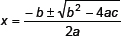# CBSE Class 10 Math, Arithmetic Progression

Class X Math
A Polynomial of the form p(x) = ax2 + bx + c, where a 0 and a, b, c are real numbers and x is a real variable is called a quadratic polynomial.
An equation p(x) = 0, where p(x) is a quadratic polynomial is called a quadratic equation i.e. ax2 + bx + c = 0, a 0.
Those values of x for which ax2 + bx + c = 0 is satisfied are called zeros of quadratic equation.
Quadratic equation is classified into two categories
ax2 + c = 0
by putting b = 0 in ax2 + bx + c = 0
Affected quadratic equation of type ax2 + bx + c = 0, b 0.
If α, β are the zeros of the polynomial ax2 + bx + c. Then α, β are called roots of corresponding equation
ax2 + bx + c = 0
⇒        p(α) = p(β) = 0
i.e. 2 + + c = 0
and          aβ2 + bβ + c = 0
Pure quadratic ax2 + c = 0 can be solved by any one of the following methods:
By Taking square root
By factorisation
Affected quadratic equation can be solved by any one of the following method:
By splitting middle term
By method of completing the square
D = b2 – 4ac, is called the discriminant which decides the nature of roots.
If D > 0, Roots are real and unequal.
If D = 0, Roots are real and equal.
If D < 0, No Real roots are possible.
The quadratic formula or Sridharacharya’s formula to find the roots of ax2 + bx + c = 0 is